## Eigenvalue

Letbe a linear transformation represented by a Matrix A. If there is a Vector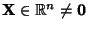such that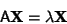(1)

for some Scalar, thenis the eigenvalue of A with corresponding (right) Eigenvector. Letting A be aMatrix,(2)

with eigenvalue, then the corresponding Eigenvectors satisfy(3)

which is equivalent to the homogeneous system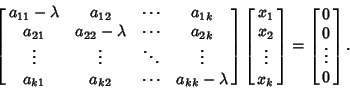(4)

Equation (4) can be written compactly as(5)

where I is the Identity Matrix.

As shown in Cramer's Rule, a system of linear equations has nontrivial solutions only if the Determinant vanishes, so we obtain the Characteristic Equation(6)

If alls are different, then plugging these back in givesindependent equations for thecomponents of each corresponding Eigenvector. The Eigenvectors will then be orthogonal and the system is said to be nondegenerate. If the eigenvalues are-fold Degenerate, then the system is said to be degenerate and the Eigenvectors are not linearly independent. In such cases, the additional constraint that the Eigenvectors be orthogonal,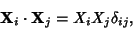(7)

whereis the Kronecker Delta, can be applied to yieldadditional constraints, thus allowing solution for the Eigenvectors.

Assume A has nondegenerate eigenvalues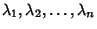and corresponding linearly independent Eigenvectorswhich can be denoted(8)

Define the matrices composed of eigenvectors(9)

and eigenvalues(10)

where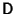is a Diagonal Matrix. Then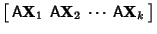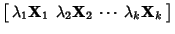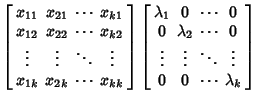(11)

so(12)

Furthermore,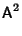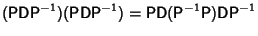(13)

By induction, it follows that for,(14)

The inverse of A is(15)

where the inverse of the Diagonal Matrix D is trivially given by(16)

Equation (14) therefore holds for both Positive and Negative.

A further remarkable result involving the matricesandfollows from the definition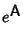(17)

Since D is a Diagonal Matrix,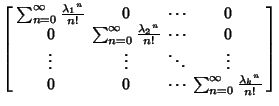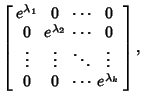(18)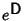can be found using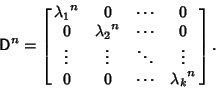(19)

Assume we know the eigenvalue for(20)

Adding a constant times the Identity Matrix to A,(21)

so the new eigenvalues equal the old plus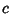. Multiplying A by a constant(22)

so the new eigenvalues are the old multiplied by.

Now consider a Similarity Transformation of A. Letbe the Determinant of A, then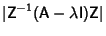(23)

so the eigenvalues are the same as for A.

See also Brauer's Theorem, Condition Number, Eigenfunction, Eigenvector, Frobenius Theorem, Gersgorin Circle Theorem, Lyapunov's First Theorem, Lyapunov's Second Theorem, Ostrowski's Theorem, Perron's Theorem, Perron-Frobenius Theorem, Poincaré Separation Theorem, Random Matrix, Schur's Inequalities, Sturmian Separation Theorem, Sylvester's Inertia Law, Wielandt's Theorem

References

Arfken, G. Eigenvectors, Eigenvalues.'' §4.7 in Mathematical Methods for Physicists, 3rd ed. Orlando, FL: Academic Press, pp. 229-237, 1985.

Nash, J. C. The Algebraic Eigenvalue Problem.'' Ch. 9 in Compact Numerical Methods for Computers: Linear Algebra and Function Minimisation, 2nd ed. Bristol, England: Adam Hilger, pp. 102-118, 1990.

Press, W. H.; Flannery, B. P.; Teukolsky, S. A.; and Vetterling, W. T. Eigensystems.'' Ch. 11 in Numerical Recipes in FORTRAN: The Art of Scientific Computing, 2nd ed. Cambridge, England: Cambridge University Press, pp. 449-489, 1992.